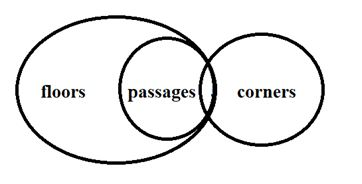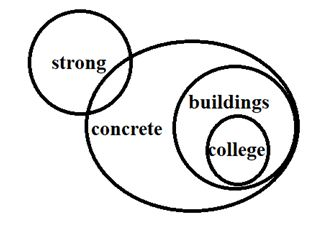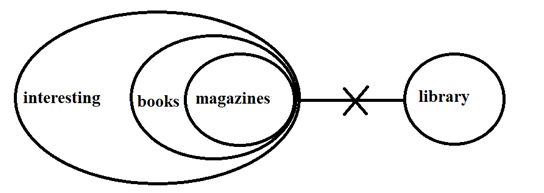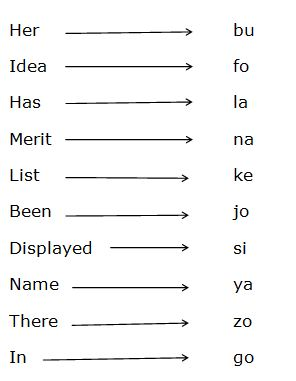# SBI Clerk Pre Reasoning (Day-46)

Dear Aspirants, Our IBPS Guide team is providing new series of Reasoning Questions for SBI Clerk Prelims 2020 so the aspirants can practice it on a daily basis. These questions are framed by our skilled experts after understanding your needs thoroughly. Aspirants can practice these new series questions daily to familiarize with the exact exam pattern and make your preparation effective.

Start Quiz

Ensure Your Ability Before the Exam – Take SBI Clerk 2020 Prelims Free Mock Test

Inequality:

Directions (1-3): Read both the conclusions and then decide which of the given conclusion logically follows from the given statements.

a) If Only conclusion I follows.

b) If Only conclusion II follows.

c) If either conclusion I or II follows.

d) If neither conclusion I nor II follows.

e) If both conclusions I and II follow.

1) Statements:

F < R ≥ O = M ≤ T = K

Conclusions:

I)K ≥ O

II) F < M

2) Statements:

G = N ≤ O ≥ P > Q = R

Conclusions:

I)O > R

II)P ≤ G

3) Statements:

F < O = L ≥ W = S

Conclusions:

I) W ≤ F

II)O ≥ S

Syllogism:

Directions (4-6): Read both the conclusions and then decide which of the given conclusion logically follows from the given statements disregarding the commonly known facts.

a) If Only conclusion I follows.

b) If Only conclusion II follows.

c) If Either conclusion I or II follows.

d) If Neither conclusion I nor II follows.

e) If Both conclusions I and II follow.

4) Statements:

All passages are floors

Some passages are corners

Some floors are not passages

Conclusions:

I) Some corners can be floors.

II) No floors are passages.

5) Statements:

All buildings are concrete

Some concrete are strong

All colleges are buildings

Conclusions:

I) All colleges can be strong is a possibility

II) Some concrete are not strong.

6) Statements:

All books are interesting

All magazines are books

No library is interesting

Conclusions:

I) Some magazines are library

II) Some books can be library.

Coding and decoding

Directions (7-10): Study the following information carefully and answer the questions below.

In a certain code language,

“Her idea has merit” is written as “fo la bu na”

“merit list has been displayed” is written as “joke la si na”

“her name displayed there” is written as “ya si bu zo”

“name in merit list” is written as “na ya go ke”

Note: All the codes are in two letter format

7) What does “ke” stands for?

a) Been

b) Has

c) Merit

d) Name

e) List

8) What is the code for “idea”?

a) Fo

b) La

c) Bu

d) Na

e) Either Bu or Na

9) Which of the following represents “name has been displayed”?

a) Ya la ke si

b) Jo si ya la

c) Si jo ke na

d) Bu ya ke la

e) Ya si jo zo

10) What does “zo” stands for?

a) There

b) Displayed

c) Name

d) Her

e) Cannot be determined.

Directions (1-3):

I) K ≥ O (O = M ≤ T = K) –> True

II) F < M (F < R ≥ O = M) –> False

So, only conclusion I follow.

I) O > R (O ≥ P > Q = R) –> True

II) P ≤ G (G = N ≤ O ≥ P) –> False

So, only conclusion I follow.

I) W ≤ F (F < O = L ≥ W) –> False

II) O ≥ S (O = L ≥ W = S) –> True

So, only conclusion II follows.’

Directions (4-6):So, neither conclusion I nor II follows.So, only conclusion I follow.So, neither conclusion I nor II follows.

Directions (7-10):